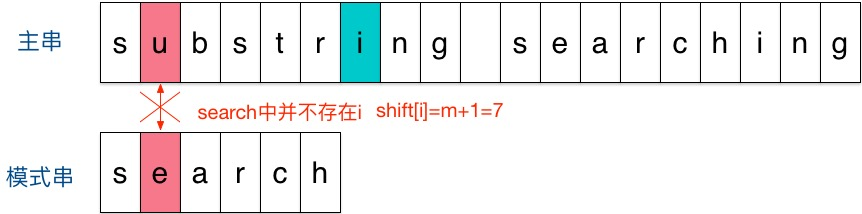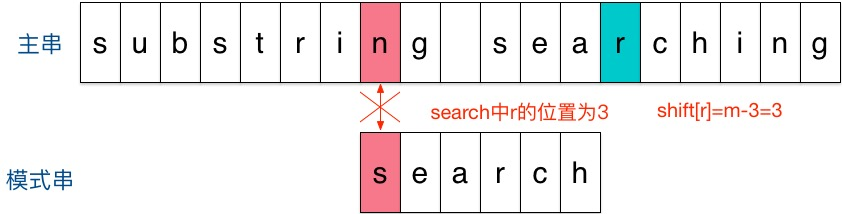# 字符串匹配——Sunday算法ACM 同时被 2 个专栏收录151 篇文章 1 订阅

# 字符串匹配——Sunday算法

## 基本思想及举例

Sunday算法由Daniel M.Sunday在1990年提出，它的思想跟BM算法很相似：1

• 如果该字符没有在模式串中出现则直接跳过，即移动位数 = 模式串长度 + 1；
• 否则，其移动位数 = 模式串长度 - 该字符最右出现的位置(以0开始) = 模式串中该字符最右出现的位置到尾部的距离 + 1。

• 刚开始时，把模式串与文主串左边对齐：• 结果发现在第2个字符处发现不匹配，不匹配时关注主串中参加匹配的最末位字符的下一位字符，即标粗的字符 i，因为模式串search中并不存在i，所以模式串直接跳过一大片，向右移动位数 = 匹配串长度 + 1 = 6 + 1 = 7，从 i 之后的那个字符（即字符n）开始下一步的匹配，如下图：• 结果第一个字符就不匹配，再看主串中参加匹配的最末位字符的下一位字符，是’r’，它出现在模式串中的倒数第3位，于是把模式串向右移动3位（m - 3 = 6 - 3 = r 到模式串末尾的距离 + 1 = 2 + 1 =3），使两个’r’对齐，如下：• 匹配成功。

回顾整个过程，我们只移动了两次模式串就找到了匹配位置，缘于Sunday算法每一步的移动量都比较大，效率很高。

## 偏移表

shift[w]={mmax{i<m|P[i]=w}m+1 if w is in P[0..m1] otherwise

m = 6
shift[s] = 6 - max(s的位置) = 6 - 0 = 6
shift[e] = 6 - max(e的位置) = 6 - 1 = 5
shift[a] = 6 - max(a的位置) = 6 - 2 = 4
shift[r] = 6 - max(r的位置) = 6 - 3 = 3
shift[c] = 6 - max(c的位置) = 6 - 4 = 2
shift[h] = 6 - max(h的位置) = 6 - 5 = 1
shift[其他] = m + 1 = 6 + 1 = 7

## 伪代码

Sunday(T, P)
01 n <- the length of T
02 m <- the length of P
03 // computer the shift table for P
04 for c <- 0 to the length of OffsetTable - 1
05  shift[c] = m + 1
06 for k <- 0 to m - 1
07  shift[P[k]] = m - k
08 // search
09 s <- 0
10 while s ≤ n - m do
11  j <- 0  // start from the begin
12  // check if T[s..s+m-1] = P[0..m-1]
13  while T[s + j] = P[j] do
14      j <- j + 1
15      if j ≥ m then return s
16  s <- s + shift[T[s + m]]
17 return -1

## 算法实现

const int maxNum = 1005;
int shift[maxNum];
int Sunday(const string& T, const string& P) {
int n = T.length();
int m = P.length();

// 默认值，移动m+1位
for(int i = 0; i < maxNum; i++) {
shift[i] = m + 1;
}

// 模式串P中每个字母出现的最后的下标
// 所对应的主串参与匹配的最末位字符的下一位字符移动到该位，所需要的移动位数
for(int i = 0; i < m; i++) {
shift[P[i]] = m - i;
}

// 模式串开始位置在主串的哪里
int s = 0;
// 模式串已经匹配到的位置
int j;
while(s <= n - m) {
j = 0;
while(T[s + j] == P[j]) {
j++;
// 匹配成功
if(j >= m) {
return s;
}
}
// 找到主串中当前跟模式串匹配的最末字符的下一个字符
// 在模式串中出现最后的位置
// 所需要从(模式串末尾+1)移动到该位置的步数
s += shift[T[s + m]];
}
return -1;
}

## 测试主程序

#include <iostream>
#include <string>

using namespace std;

const int maxNum = 1005;

int shift[maxNum];
int Sunday(const string& T, const string& P) {
int n = T.length();
int m = P.length();

// 默认值，移动m+1位
for(int i = 0; i < maxNum; i++) {
shift[i] = m + 1;
}

// 模式串P中每个字母出现的最后的下标
// 所对应的主串参与匹配的最末位字符的下一位字符移动到该位，所需要的移动位数
for(int i = 0; i < m; i++) {
shift[P[i]] = m - i;
}

// 模式串开始位置在主串的哪里
int s = 0;
// 模式串已经匹配到的位置
int j;
while(s <= n - m) {
j = 0;
while(T[s + j] == P[j]) {
j++;
// 匹配成功
if(j >= m) {
return s;
}
}
// 找到主串中当前跟模式串匹配的最末字符的下一个字符
// 在模式串中出现最后的位置
// 所需要从(模式串末尾+1)移动到该位置的步数
s += shift[T[s + m]];
}
return -1;
}

/**
IN
at the thought of
though

OUT
7
**/
int main() {
// 主串和模式串
string T, P;
while(true) {
// 获取一行
getline(cin, T);
getline(cin, P);
int res = Sunday(T, P);
if(res == -1) {
cout << "主串和模式串不匹配。" << endl;
} else {
cout << "模式串在主串的位置为：" << res << endl;
}
}
return 0;
}

## 输出数据

at the thought of
though

abcd
d

asd
d

gf

gagewgwe
wefgwef

gwagweg
g

gergregeagbb
bb

## 算法分析2

• Sunday预处理阶段的时间为：O( $\left| \begin{matrix} \sum \end{matrix} \right|$ + m)

• 最坏情况下时间复杂度为：O(nm)

• 平均时间复杂度：O(n)

• 空间复杂度：O( $\left| \begin{matrix} \sum \end{matrix} \right|$)

04-23
05-0404-181万+
02-297935
01-013453
01-113078
10-17584
10-08
03-25
05-17107
02-1155
09-05
09-141万+
05-13361
12-08437
08-03507
10-24点击重新获取扫码支付余额充值